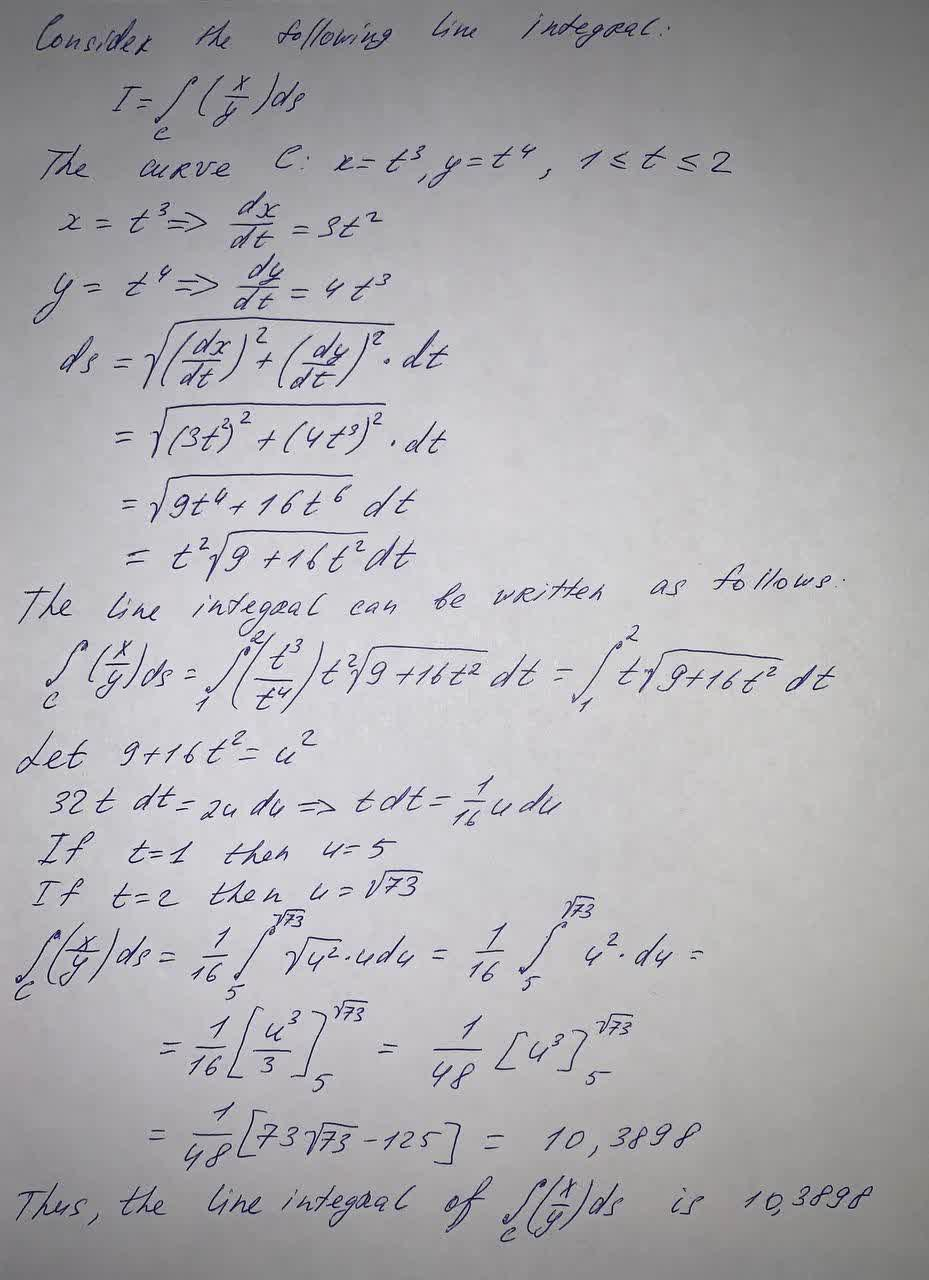Question# Evaluate the line integral, where C is the given curve C (\frac{x}{y})ds, C: x = t^3, y = t^4, 1 \leq t \leq 2

Integrals
ANSWEREDC $$(\frac{x}{y})ds, C: x = t^3, y = t^4, 1 \leq t \leq 2$$